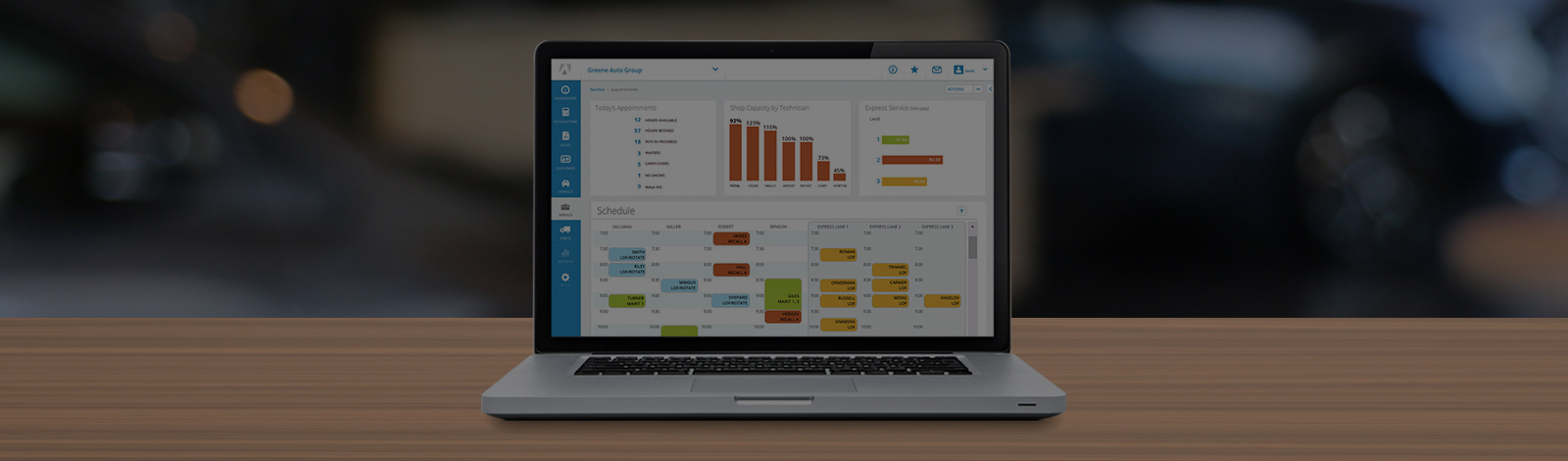FlexDMS Help has moved here. This site will close on November 18th.

# Creating the Cost-Plus Table for Retail Matrix Pricing

Autosoft -

Use the Retail Matrix table to establish up to 12 different levels to adjust pricing for any given retail matrix set. Many dealers have found that matrix pricing is more profitable for repair order parts sales at the lower end of the scale. You can use a modified form of matrix pricing by entering only one or two levels.

To learn how to access the Retail Pricing Matrix screen, see the Knowledge Base article, "Adding a Retail Matrix Pricing Level."### Markup vs. Margin

Before you can build your Cost-Plus table, you need to do a few calculations. Retail matrix pricing is calculated using markup or a cost-plus strategy. However, many dealers want to achieve sales with a guaranteed profit margin. This is the gross profit expressed as a percent of the sale calculated using the following equation.

(Sale Price - Cost) ÷ Sale Price X 100 = Margin%

Because the cost is set by the supplier, you must determine what the retail sale price needs to be in order to achieve a particular margin. You can determine the selling price by using the following formula.

100 – Desired% = Factor

Example:  100 – 40 = 60

Once the “Factor” is determined, you can calculate the required sale price using the following formula.

(Cost ÷ Factor) X 100 = Sale Price

Example:  (\$1.00 ÷ 60) X 100 = \$1.67

Once you know the required sale price, you must determine how much the cost needs to be marked up to achieve this price. Use the following formula to determine the markup percent.

(Sale Price – Cost) ÷ Cost X 100 = Markup%

Example:  (\$1.67 – \$1.00) ÷ \$1.00 X 100 = 67

This is the profit expressed as a percent of the cost. The Markup% is what you enter in the Add field in the Retail Matrix.

Once you have determined the desired percentage of gross profit for your established groups of parts and, in turn, the percentage markup required to achieve that gross profit, you can enter the levels in the Cost-Plus table.

### Building the Cost Plus Table

In the Up To fields of the Retail Matrix table, type the maximum dollar amount for which the markup percentage applies. This represents the upper limit of the level. In the Add fields, type the markup percent for the level.

For example, if the Up To amount for level 1 is \$5.00 and the Add percentage is 500%, any part with a cost under \$5.00 will be marked up at five times its cost (which is cost plus five times cost). The selling price for a part with a cost of \$5.00 or higher would default to one of the following:

• A markup from cost using the next applicable level,
• A suggested retail price as calculated from any applicable up charges below the matrix table on this screen, or
• The list price from the OEM price tape (if neither of the first two possibilities apply).
 Tip:  If the Up To amount in level 12 is \$9999.99 with a given percentage, the Suggested Retail Plus fields are overridden.